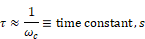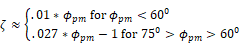### System characteristics

In designing each compensator, the following system characteristics hold true:

LM = Log magnitude, base 10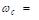Cross-over frequency of open-loop Bode LM plot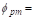Phase margin of open-loop Bode phase plot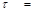Time constant, s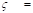Damping ratio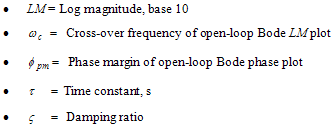The open-loop Bode frequency response can be used to approximate closed-loop system behavior, provided the open-loop frequency response satisfies three conditions:

The gain at low frequencies is relatively high for low steady-state errors

The gain at high frequencies is relatively low for disturbance attenuation

The slope of the Bode plot is approximately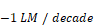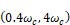When the above conditions are approximately satisfied, the following correlations are valid: